# JDK源码分析之hashmap就这么简单理解

### 一、HashMap概述

HashMap是基于哈希表的Map接口实现，此实现提供所有可选的映射操作，并允许使用null值和null键。HashMap与HashTable的作用大致相同，但是它不是线程安全的。此类不保证映射的顺序，特别是它不保证该顺序恒久不变。

### 二、HashMap的介绍

1.HashMap是存储键值对（key，value）的一种数据结构。
2.每一个元素都是一个key-value。
3.HashMap最多只允许一个key为null，允许多个key的value值为null。
4.HashMap是非线程安全的，只适用于单线程环境。
5.HashMap实现了Serializable、Cloneable接口，因此它支持序列化和克隆。

### 二、HashMap在JDK1.7和JDK1.8的区别

JDK1.7的HashMap是基于一个数组加多个单链表来实现的，hash值冲突时，就将对应节点以链表的形式存储，这样子HashMap在性能上就存在一定的问题，为什么这么说呢？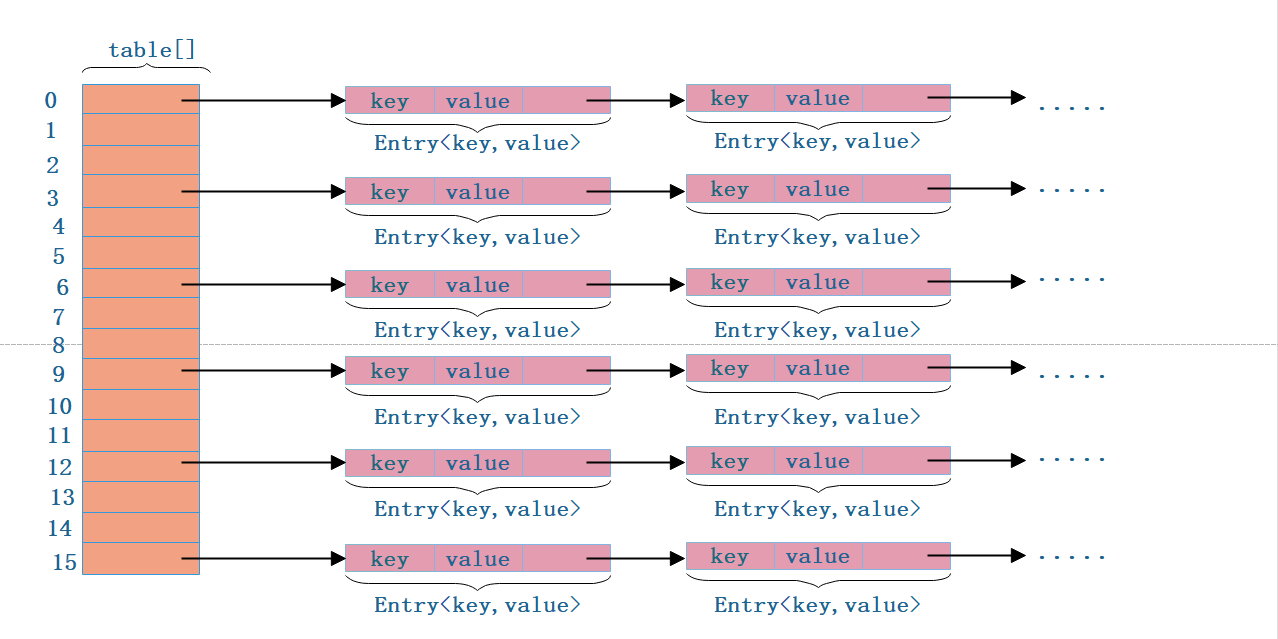/** 初始容量，默认16 */   (1)
static final int DEFAULT_INITIAL_CAPACITY = 1 << 4; // aka 16

/** 最大初始容量，2^30 */  (2)
static final int MAXIMUM_CAPACITY = 1 << 30;

/** 负载因子，默认0.75，负载因子越小，hash冲突机率越低 */  (3)
static final float DEFAULT_LOAD_FACTOR = 0.75f;

/** 初始化一个Entry的空数组 */  (4)
static final Entry<?,?>[] EMPTY_TABLE = {};

/** 将初始化好的空数组赋值给table，table数组是HashMap实际存储数据的地方，并不在EMPTY_TABLE数组中 */ (5)
transient Entry<K,V>[] table = (Entry<K,V>[]) EMPTY_TABLE;

/** HashMap实际存储的元素个数 */ (6)
transient int size;

/** 临界值（HashMap 实际能存储的大小）,公式为(threshold = capacity * loadFactor) */ (7)
int threshold;

/** 负载因子 */ (8)

/** HashMap的结构被修改的次数，用于迭代器 */ (9)
transient int modCount;

 　　//计算Hash值时的key
transient int hashSeed = 0;

//通过初始容量和状态因子构造HashMap
public HashMap(int initialCapacity, float loadFactor) {　　　　　//（1）
if (initialCapacity < 0)//参数有效性检查
throw new IllegalArgumentException("Illegal initial capacity: " + initialCapacity);　　　　　//（2）
if (initialCapacity > MAXIMUM_CAPACITY)//参数有效性检查
initialCapacity = MAXIMUM_CAPACITY; 　　　　　//（3）
throw new IllegalArgumentException("Illegal load factor: " +

threshold = initialCapacity;　　　　　//(4)
init();
}

//(5)
public HashMap(int initialCapacity) {
}

//（6）
public HashMap() {
}

//（7）
public HashMap(Map<? extends K, ? extends V> m) {
inflateTable(threshold); 　　　　//（9）
putAllForCreate(m);
} 

transient Entry<K,V>[] table = (Entry<K,V>[]) EMPTY_TABLE;

// 静态内部类
static class Entry<K,V> implements Map.Entry<K,V> {　　　　　//(1)
final K key;　　　　　//(2)
V value;　　　　　//(3)
Entry<K,V> next; // 只想下一个entry节点　　　　　//(4)
int hash;

/**
* 构造函数，每次都用新的节点指向链表的头结点。新节点作为链表新的头结点
*/
Entry(int h, K k, V v, Entry<K,V> n) {
value = v;
next = n; // !!!
key = k;
hash = h;
}

public final K getKey() {
return key;
}

public final V getValue() {
return value;
}

public final V setValue(V newValue) {
V oldValue = value;
value = newValue;
return oldValue;
}

public final boolean equals(Object o) {
if (!(o instanceof Map.Entry))
return false;
Map.Entry e = (Map.Entry)o;
Object k1 = getKey();
Object k2 = e.getKey();
if (k1 == k2 || (k1 != null && k1.equals(k2))) {
Object v1 = getValue();
Object v2 = e.getValue();
if (v1 == v2 || (v1 != null && v1.equals(v2)))
return true;
}
return false;
}

public final int hashCode() {
return (key==null   ? 0 : key.hashCode()) ^
(value==null ? 0 : value.hashCode());
}

public final String toString() {
return getKey() + "=" + getValue();
}

//(5)
void recordAccess(HashMap<K,V> m) {
}

//(6)
void recordRemoval(HashMap<K,V> m) {
}
}

#### 1.put 方法 源码如下：

   public V put(K key, V value) {　　　　　//(1)
if (table == EMPTY_TABLE) {
inflateTable(threshold);
}　　　　　//（2）
if (key == null)
return putForNullKey(value);　　　　　//(3)
int hash = hash(key);　　　　　//(4)
int i = indexFor(hash, table.length);　　　　　//(5)
for (Entry<K,V> e = table[i]; e != null; e = e.next) {
Object k;　　　　　　　//(6)
if (e.hash == hash && ((k = e.key) == key || key.equals(k))) {
V oldValue = e.value;
e.value = value;
e.recordAccess(this);
return oldValue;
}
}
//(7)
modCount++;　　　　　//(8)
return null;
}

　　private void inflateTable(int toSize) {
int capacity = roundUpToPowerOf2(toSize);//（1）
threshold = (int) Math.min(capacity * loadFactor, MAXIMUM_CAPACITY + 1);//（2）
table = new Entry[capacity];//（3）
initHashSeedAsNeeded(capacity);//（4）
}

inflateTable这个方法用于为主干数组table在内存中分配存储空间，通过roundUpToPowerOf2(toSize)可以确保capacity为大于或等于toSize的最接近toSize的二次幂，比如toSize=13,则capacity=16；to_size=16，capacity=16；to_size=17,capacity=32。roundUpToPowerOf2(toSize)源码如下所示：

　　private static int roundUpToPowerOf2(int number) {
// assert number >= 0 : "number must be non-negative";
return number >= MAXIMUM_CAPACITY
? MAXIMUM_CAPACITY
: (number > 1) ? Integer.highestOneBit((number - 1) << 1) : 1;
}

roundUpToPowerOf2中的这段处理使得数组长度一定为2的次幂，Integer.highestOneBit是用来获取最左边的bit（其他bit位为0）所代表的数值。

　　//(1)
final int hash(Object k) { 　　　　　//(2)
int h = hashSeed;　　　　　//(3)
if (0 != h && k instanceof String) {//这里针对String优化了Hash函数，是否使用新的Hash函数和Hash因子有关
return sun.misc.Hashing.stringHash32((String) k);
}
//(4)
h ^= k.hashCode();
//(5)
h ^= (h >>> 20) ^ (h >>> 12);
return h ^ (h >>> 7) ^ (h >>> 4);
}
h ^= k.hashCode(): 0101 1101 0010 1111 1100 0110 0011 0101
------------------------------------------------------------
h >>> 20：       : 0000 0000 0000 0000 0000 0101 1101 0010
h >>> 12         : 0000 0000 0000 0101 1101 0010 1111 1100
------------------------------------------------------------
(h >>> 20) ^ (h >>> 12)
: 0000 0000 0000 0101 1101 0111 0010 1110
-----------------------------------------------------------
h ^= (h >>> 20) ^ (h >>> 12)
: 0101 1101 0010 1010 0001 0001 0001 1011
-----------------------------------------------------------
(h >>> 7)        : 0000 0000 1011 1010 0101 0100 0010 0010
(h >>> 4)        : 0000 0101 1101 0010 1010 0001 0001 0001
-----------------------------------------------------------
(h >>> 7) ^ (h >>> 4)
：0000 0101 0110 1000 1111 0101 0011 0011
-----------------------------------------------------------
h ^ (h >>> 7) ^ (h >>> 4)
：0101 1000 0100 0010 1110 0100 0010 1000
-----------------------------------------------------------
h & (length-1)   ：0000 0000 0000 0000 0000 0000 0000 1000   = 8

 　　//返回数组下标
static int indexFor(int h, int length) {　　　　//(1)
return h & (length-1);
}

    10100101 11000100 00100101
&   00000000 00000000 00001111
----------------------------------
00000000 00000000 00000101 //高位全部归零，只保留末四位

 void addEntry(int hash, K key, V value, int bucketIndex) {　　　　　//(1)
if ((size >= threshold) && (null != table[bucketIndex])) {　　　　　　　//(2)
resize(2 * table.length);　　　　　　　//(3)
hash = (null != key) ? hash(key) : 0;　　　　　　　//(4)
bucketIndex = indexFor(hash, table.length);
}
//（5）
createEntry(hash, key, value, bucketIndex);
}

void createEntry(int hash, K key, V value, int bucketIndex) {　　　　　//(6)
Entry<K,V> e = table[bucketIndex];　　　　　//(7)
table[bucketIndex] = new Entry<>(hash, key, value, e);　　　　　//元素个数加1
size++;
}

　　//按新的容量扩容Hash表
void resize(int newCapacity) {　　　　　//(1)
Entry[] oldTable = table;　　　　　//(2)
int oldCapacity = oldTable.length;　　　　　//(3)
if (oldCapacity == MAXIMUM_CAPACITY) {　　　　　　　//(4)
threshold = Integer.MAX_VALUE;//修改扩容阀值
return;
}
//(5)
Entry[] newTable = new Entry[newCapacity];　　　　 boolean oldAltHashing = useAltHashing; 　　　　 //(6)计算是否需要对键重新进行哈希码的计算　　　　 useAltHashing |= sun.misc.VM.isBooted() && (newCapacity >= Holder.ALTERNATIVE_HASHING_THRESHOLD);　　　　 boolean rehash = oldAltHashing ^ useAltHashing;　　　　　//（7）
transfer(newTable, rehash);　　　　　//(8)
table = newTable;　　　　　//(9)
threshold = (int)Math.min(newCapacity * loadFactor, MAXIMUM_CAPACITY + 1);
} 

　　void transfer(Entry[] newTable, boolean rehash) {
int newCapacity = newTable.length;
//（1）
for (Entry<K,V> e : table) {
while(null != e) {
Entry<K,V> next = e.next;　　　　　　　　　　//（2）
if (rehash) {
//(3)
e.hash = null == e.key ? 0 : hash(e.key);
}
//（4）
int i = indexFor(e.hash, newCapacity);
//（5）
e.next = newTable[i];
//（6）
newTable[i] = e;　　　　　　　　　 //(7)
e = next;
}
}
}

  　 //(1)　　 public V get(Object key) {　　　　　//(2）
if (key == null)
return getForNullKey();　　　　　//(3)
Entry<K,V> entry = getEntry(key);
//(4)
return null == entry ? null : entry.getValue();
}

final Entry<K,V> getEntry(Object key) {　　　　　//(5)
if (size == 0) {
return null;
}
//（6）
int hash = (key == null) ? 0 : hash(key);　　　　　//(7)
for (Entry<K,V> e = table[indexFor(hash, table.length)];
e != null;
e = e.next) {
Object k;　　　　　　　//(8)
if (e.hash == hash &&
((k = e.key) == key || (key != null && key.equals(k))))
return e;
}　　　　　//(9)
return null;
}
　　 //获取key为null的实体
private V getForNullKey() {
if (size == 0) {//如果元素个数为0，则直接返回null
return null;
}
//key为null的元素存储在table的第0个位置
for (Entry<K,V> e = table; e != null; e = e.next) {
if (e.key == null)//判断是否为null
return e.value;//返回其值
}
return null;
} 

final Entry<K,V> removeEntryForKey(Object key) {
//计算键的hash值
int hash = (key == null) ? 0 : hash(key);
//计算桶号
int i = indexFor(hash, table.length);
//记录待删除节点的上一个节点
Entry<K,V> prev = table[i];
//待删除节点
Entry<K,V> e = prev;

while (e != null) {
Entry<K,V> next = e.next;
Object k;
//是否是将要删除的节点
if (e.hash == hash &&
((k = e.key) == key || (key != null && key.equals(k)))) {
modCount++;
size--;
//将要删除的节点是否为链表的头部
if (prev == e)
//链表的头部指向下一节点
table[i] = next;
else
//上一节点的NEXT为将要删除节点的下一节点
prev.next = next;
e.recordRemoval(this);
return e;
}
prev = e;
e = next;
}

return e;
}

### JDK1.8中，HashMap的变化。

到目前为止，不知道大家有没有发现JDK1.7中需要优化的地方？

JDK1.8中的HashMap的结构图如下：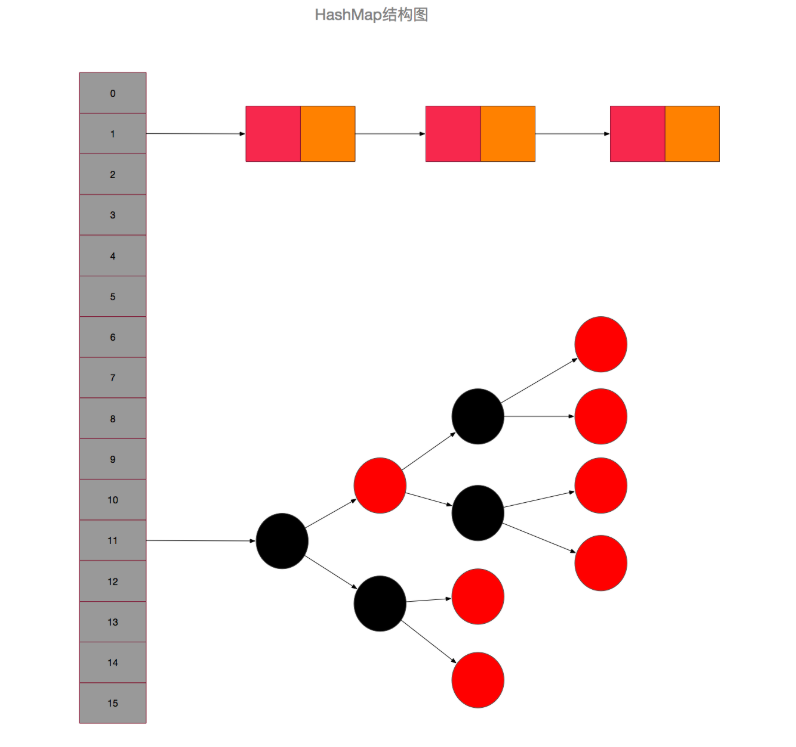//（1） 默认的初始容量是16
static final int DEFAULT_INITIAL_CAPACITY = 1 << 4;
//（2） 桶最大容量
static final int MAXIMUM_CAPACITY = 1 << 30;
//（3） 默认的负载因子
static final float DEFAULT_LOAD_FACTOR = 0.75f;
//（4） 当桶(bucket)上的结点数大于这个值时会转成红黑树
static final int TREEIFY_THRESHOLD = 8;
//（5） 当桶(bucket)上的结点数小于这个值时树转链表
static final int UNTREEIFY_THRESHOLD = 6;
//（6） 桶中结构转化为红黑树对应的table的最小大小
static final int MIN_TREEIFY_CAPACITY = 64;
//（7） 存储元素的数组，总是2的幂次倍
transient Node<k,v>[] table;
//（8） 存放具体元素的集
transient Set<map.entry<k,v>> entrySet;
//（9） 存放元素的个数，注意这个不等于数组的长度。
transient int size;
//（10） 每次扩容和更改map结构的计数器
transient int modCount;
//（11） 临界值 当实际大小(容量*填充因子)超过临界值时，会进行扩容
int threshold;
//（12） 负载因子
final float loadFactor;

public HashMap(int initialCapacity, float loadFactor) {
// 桶初始容量不能小于0，否则报错
if (initialCapacity < 0)
throw new IllegalArgumentException("Illegal initial capacity: " +
initialCapacity);
// 桶初始容量不能大于最大值，否则为最大值
if (initialCapacity > MAXIMUM_CAPACITY)
initialCapacity = MAXIMUM_CAPACITY;
//校验负载因子 负载因子不能小于或等于0，不能为非数字
throw new IllegalArgumentException("Illegal load factor: " +
// 初始化负载因子
// 初始化threshold大小
this.threshold = tableSizeFor(initialCapacity);
}

public HashMap(int initialCapacity) {
// 调用HashMap(int, float)型构造函数,通过扩容因子构造HashMap,容量取默认值，即16 。
}

public HashMap() {
// 初始化负载因子，装载因子取0.75，容量取16，构造HashMap
}
//通过其他Map来初始化HashMap,容量通过其他Map的size来计算，装载因子取0.75
public HashMap(Map<? extends K, ? extends V> m) {
// 初始化负载因子
// 将m中的所有元素添加至HashMap中
putMapEntries(m, false);
}

1.初始化threshold大小 this.threshold = tableSizeFor(initialCapacity);有所不同，tableSizeFor(initialCapacity)返回大于initialCapacity的最小的二次幂数值。源码如下：

static final int tableSizeFor(int cap) {
int n = cap - 1;
n |= n >>> 1;
n |= n >>> 2;
n |= n >>> 4;
n |= n >>> 8;
n |= n >>> 16;
return (n < 0) ? 1 : (n >= MAXIMUM_CAPACITY) ? MAXIMUM_CAPACITY : n + 1;
}

>>> 操作符表示无符号右移，高位取0。

2.通过其他Map来初始化HashMap，putMapEntries(Map<? extends K, ? extends V> m, boolean evict)函数将m的所有元素存入本HashMap实例中。源码如下：

final void putMapEntries(Map<? extends K, ? extends V> m, boolean evict) {
int s = m.size();
if (s > 0) {
// 判断table是否已经初始化
if (table == null) { // pre-size
// 未初始化，s为m的实际元素个数
float ft = ((float)s / loadFactor) + 1.0F;
int t = ((ft < (float)MAXIMUM_CAPACITY) ?
(int)ft : MAXIMUM_CAPACITY);
// 计算得到的t大于阈值，则初始化阈值
if (t > threshold)
threshold = tableSizeFor(t);
}
// 已初始化，并且m元素个数大于阈值，进行扩容处理
else if (s > threshold)
resize();
// 将m中的所有元素添加至HashMap中
for (Map.Entry<? extends K, ? extends V> e : m.entrySet()) {
K key = e.getKey();
V value = e.getValue();
putVal(hash(key), key, value, false, evict);
}
}
}

static final int hash(Object key) {
int h;
return (key == null) ? 0 : (h = key.hashCode()) ^ (h >>> 16);
}

先看put方法，如下：

final V putVal(int hash, K key, V value, boolean onlyIfAbsent,
boolean evict) {
Node<K,V>[] tab; Node<K,V> p; int n, i;
// （1）
if ((tab = table) == null || (n = tab.length) == 0)
n = (tab = resize()).length;
// （2）
if ((p = tab[i = (n - 1) & hash]) == null)
tab[i] = newNode(hash, key, value, null);
// 桶中已经存在元素
else {
Node<K,V> e; K k;
// （3）
if (p.hash == hash &&
((k = p.key) == key || (key != null && key.equals(k))))
// 将第一个元素赋值给e，用e来记录
e = p;
// （4）
else if (p instanceof TreeNode)
// 放入树中
e = ((TreeNode<K,V>)p).putTreeVal(this, tab, hash, key, value);
// 该链为链表
// 为链表结点
else {
//（5）
for (int binCount = 0; ; ++binCount) {
// 到达链表的尾部
if ((e = p.next) == null) {
// 在尾部插入新结点
p.next = newNode(hash, key, value, null);
// （6）
if (binCount >= TREEIFY_THRESHOLD - 1) // -1 for 1st
treeifyBin(tab, hash);
// 跳出循环
break;
}
// （7）
if (e.hash == hash &&
((k = e.key) == key || (key != null && key.equals(k))))
// 相等，跳出循环
break;
// 用于遍历桶中的链表，与前面的e = p.next组合，可以遍历链表
p = e;
}
}
// （8）
if (e != null) {
// 记录e的value
V oldValue = e.value;
// onlyIfAbsent为false或者旧值为null
if (!onlyIfAbsent || oldValue == null)
//用新值替换旧值
e.value = value;
// 访问后回调
afterNodeAccess(e);
// 返回旧值
return oldValue;
}
}
// 结构性修改
++modCount;
// （9）
if (++size > threshold)
resize();
// 插入后回调
afterNodeInsertion(evict);
return null;
}

HashMap的数据存储实现原理

1. 根据key计算得到key.hash = (h = k.hashCode()) ^ (h >>> 16)；

2. 根据key.hash计算得到桶数组的索引index = key.hash & (table.length - 1)，这样就找到该key的存放位置了：

① 如果该位置没有数据，用该数据新生成一个节点保存新数据，返回null；

② 如果该位置有数据是一个红黑树，那么执行相应的插入 / 更新操作；

③ 如果该位置有数据是一个链表，分两种情况一是该链表没有这个节点，另一个是该链表上有这个节点，注意这里判断的依据是key.hash是否一样：

HashMap的put会返回key的上一次保存的数据，比如：

HashMap<String, String> map = new HashMap<String, String>();
System.out.println(map.put("a", "A")); // 打印null
System.out.println(map.put("a", "AA")); // 打印A
System.out.println(map.put("a", "AB")); // 打印AA

/**
* Tree version of putVal.
* 红黑树插入会同时维护原来的链表属性, 即原来的next属性
*/
final TreeNode<K,V> putTreeVal(HashMap<K,V> map, Node<K,V>[] tab,
int h, K k, V v) {
Class<?> kc = null;
boolean searched = false;
// 查找根节点, 索引位置的头节点并不一定为红黑树的根结点
TreeNode<K,V> root = (parent != null) ? root() : this;
for (TreeNode<K,V> p = root;;) {    // 将根节点赋值给p, 开始遍历
int dir, ph; K pk;
if ((ph = p.hash) > h)  // 如果传入的hash值小于p节点的hash值
dir = -1;    // 则将dir赋值为-1, 代表向p的左边查找树
else if (ph < h)    // 如果传入的hash值大于p节点的hash值,
dir = 1;    // 则将dir赋值为1, 代表向p的右边查找树
// 如果传入的hash值和key值等于p节点的hash值和key值, 则p节点即为目标节点, 返回p节点
else if ((pk = p.key) == k || (k != null && k.equals(pk)))
return p;
// 如果k所属的类没有实现Comparable接口 或者 k和p节点的key相等
else if ((kc == null &&
(kc = comparableClassFor(k)) == null) ||
(dir = compareComparables(kc, k, pk)) == 0) {
if (!searched) {    // 第一次符合条件, 该方法只有第一次才执行
TreeNode<K,V> q, ch;
searched = true;
// 从p节点的左节点和右节点分别调用find方法进行查找, 如果查找到目标节点则返回
if (((ch = p.left) != null &&
(q = ch.find(h, k, kc)) != null) ||
((ch = p.right) != null &&
(q = ch.find(h, k, kc)) != null))
return q;
}
// 否则使用定义的一套规则来比较k和p节点的key的大小, 用来决定向左还是向右查找
dir = tieBreakOrder(k, pk); // dir<0则代表k<pk，则向p左边查找；反之亦然
}

TreeNode<K,V> xp = p;   // xp赋值为x的父节点,中间变量,用于下面给x的父节点赋值
// dir<=0则向p左边查找,否则向p右边查找,如果为null,则代表该位置即为x的目标位置
if ((p = (dir <= 0) ? p.left : p.right) == null) {
// 走进来代表已经找到x的位置，只需将x放到该位置即可
Node<K,V> xpn = xp.next;    // xp的next节点
// 创建新的节点, 其中x的next节点为xpn, 即将x节点插入xp与xpn之间
TreeNode<K,V> x = map.newTreeNode(h, k, v, xpn);
if (dir <= 0)   // 如果时dir <= 0, 则代表x节点为xp的左节点
xp.left = x;
else        // 如果时dir> 0, 则代表x节点为xp的右节点
xp.right = x;
xp.next = x;    // 将xp的next节点设置为x
x.parent = x.prev = xp; // 将x的parent和prev节点设置为xp
// 如果xpn不为空,则将xpn的prev节点设置为x节点,与上文的x节点的next节点对应
if (xpn != null)
((TreeNode<K,V>)xpn).prev = x;
moveRootToFront(tab, balanceInsertion(root, x)); // 进行红黑树的插入平衡调整
return null;
}
}
}

1.查找当前红黑树的根结点，将根结点赋值给p节点，开始进行查找

2.如果传入的hash值小于p节点的hash值，将dir赋值为-1，代表向p的左边查找树

3.如果传入的hash值大于p节点的hash值， 将dir赋值为1，代表向p的右边查找树

4.如果传入的hash值等于p节点的hash值，并且传入的key值跟p节点的key值相等, 则该p节点即为目标节点，返回p节点

5.如果k所属的类没有实现Comparable接口，或者k和p节点的key使用compareTo方法比较相等：第一次会从p节点的左节点和右节点分别调用find方法（见上文代码块2）进行查找，如果查找到目标节点则返回；如果不是第一次或者调用find方法没有找到目标节点，则调用tieBreakOrder方法（见下文代码块5）比较k和p节点的key值的大小，以决定向树的左节点还是右节点查找。

6.如果dir <= 0则向左节点查找（p赋值为p.left，并进行下一次循环），否则向右节点查找，如果已经无法继续查找（p赋值后为null），则代表该位置即为x的目标位置，另外变量xp用来记录查找的最后一个节点，即下文新增的x节点的父节点。

7.以传入的hash、key、value参数和xp节点的next节点为参数，构建x节点（注意：xp节点在此处可能是叶子节点、没有左节点的节点、没有右节点的节点三种情况，即使它是叶子节点，它也可能有next节点，红黑树的结构跟链表的结构是互不影响的，不会因为某个节点是叶子节点就说它没有next节点，红黑树在进行操作时会同时维护红黑树结构和链表结构，next属性就是用来维护链表结构的），根据dir的值决定x决定放在xp节点的左节点还是右节点，将xp的next节点设为x，将x的parent和prev节点设为xp，如果原xp的next节点（xpn）不为空, 则将该节点的prev节点设置为x节点, 与上面的将x节点的next节点设置为xpn对应。

8.进行红黑树的插入平衡调整，

final void treeifyBin(Node<K,V>[] tab, int hash) {
int n, index; Node<K,V> e;
// table为空或者table的长度小于64, 进行扩容
if (tab == null || (n = tab.length) < MIN_TREEIFY_CAPACITY)
resize();
// 根据hash值计算索引值, 遍历该索引位置的链表
else if ((e = tab[index = (n - 1) & hash]) != null) {
TreeNode<K,V> hd = null, tl = null;
do {
TreeNode<K,V> p = replacementTreeNode(e, null); // 链表节点转红黑树节点
if (tl == null)    // tl为空代表为第一次循环
hd = p; // 头结点
else {
p.prev = tl;    // 当前节点的prev属性设为上一个节点
tl.next = p;    // 上一个节点的next属性设置为当前节点
}
tl = p; // tl赋值为p, 在下一次循环中作为上一个节点
} while ((e = e.next) != null);    // e指向下一个节点
// 将table该索引位置赋值为新转的TreeNode的头节点
if ((tab[index] = hd) != null)
hd.treeify(tab);    // 以头结点为根结点, 构建红黑树
}

1.校验table是否为空，如果长度小于64，则调用resize方法（见下文resize方法）进行扩容。

2.根据hash值计算索引值，将该索引位置的节点赋值给e节点，从e节点开始遍历该索引位置的链表。

3.调用replacementTreeNode方法（该方法就一行代码，直接返回一个新建的TreeNode）将链表节点转为红黑树节点，将头结点赋值给hd节点，每次遍历结束将p节点赋值给tl，用于在下一次循环中作为上一个节点进行一些链表的关联操作（p.prev = tl 和 tl.next = p）。

4.将table该索引位置赋值为新转的TreeNode的头节点hd，如果该节点不为空，则以hd为根结点，调用treeify方法（见下文代码块7）构建红黑树。

final void treeify(Node<K,V>[] tab) {   // 构建红黑树
TreeNode<K,V> root = null;
for (TreeNode<K,V> x = this, next; x != null; x = next) {// this即为调用此方法的TreeNode
next = (TreeNode<K,V>)x.next;   // next赋值为x的下个节点
x.left = x.right = null;    // 将x的左右节点设置为空
if (root == null) { // 如果还没有根结点, 则将x设置为根结点
x.parent = null;    // 根结点没有父节点
x.red = false;  // 根结点必须为黑色
root = x;   // 将x设置为根结点
}
else {
K k = x.key;    // k赋值为x的key
int h = x.hash;    // h赋值为x的hash值
Class<?> kc = null;
// 如果当前节点x不是根结点, 则从根节点开始查找属于该节点的位置
for (TreeNode<K,V> p = root;;) {
int dir, ph;
K pk = p.key;
if ((ph = p.hash) > h)  // 如果x节点的hash值小于p节点的hash值
dir = -1;   // 则将dir赋值为-1, 代表向p的左边查找
else if (ph < h)    // 与上面相反, 如果x节点的hash值大于p节点的hash值
dir = 1;    // 则将dir赋值为1, 代表向p的右边查找
// 走到这代表x的hash值和p的hash值相等，则比较key值
else if ((kc == null && // 如果k没有实现Comparable接口 或者 x节点的key和p节点的key相等
(kc = comparableClassFor(k)) == null) ||
(dir = compareComparables(kc, k, pk)) == 0)
// 使用定义的一套规则来比较x节点和p节点的大小，用来决定向左还是向右查找
dir = tieBreakOrder(k, pk);

TreeNode<K,V> xp = p;   // xp赋值为x的父节点,中间变量用于下面给x的父节点赋值
// dir<=0则向p左边查找,否则向p右边查找,如果为null,则代表该位置即为x的目标位置
if ((p = (dir <= 0) ? p.left : p.right) == null) {
x.parent = xp;  // x的父节点即为最后一次遍历的p节点
if (dir <= 0)   // 如果时dir <= 0, 则代表x节点为父节点的左节点
xp.left = x;
else    // 如果时dir > 0, 则代表x节点为父节点的右节点
xp.right = x;
// 进行红黑树的插入平衡(通过左旋、右旋和改变节点颜色来保证当前树符合红黑树的要求)
root = balanceInsertion(root, x);
break;
}
}
}
}
moveRootToFront(tab, root); // 如果root节点不在table索引位置的头结点, 则将其调整为头结点
}


1.从调用此方法的节点作为起点，开始进行遍历，并将此节点设为root节点，标记为黑色（x.red = false）。

2.如果当前节点不是根结点，则从根节点开始查找属于该节点的位置（该段代码跟之前的代码块2和代码块4的查找代码类似）。

3.如果x节点(将要插入红黑树的节点)的hash值小于p节点(当前遍历到的红黑树节点)的hash值，则向p节点的左边查找。

4.与3相反，如果x节点的hash值大于p节点的hash值，则向p节点的右边查找。

5.如果x的key没有实现Comparable接口，或者x节点的key和p节点的key相等，使用tieBreakOrder方法（见上文代码块5）来比较x节点和p节点的大小，以决定向左还是向右查找（dir <= 0向左，否则向右）。

6.如果dir <= 0则向左节点查找（p赋值为p.left，并进行下一次循环），否则向右节点查找，如果已经无法继续查找（p赋值后为null），则代表该位置即为x的目标位置，另外变量xp用来记录最后一个节点，即为下文新增的x节点的父节点。

7.将x的父节点设置为xp，根据dir的值决定x决定放在xp节点的左节点还是右节点，最后进行红黑树的插入平衡调整。

8.调用moveRootToFront方法（如下：）将root节点调整到索引位置的头结点。

/**
* 如果当前索引位置的头节点不是root节点, 则将root的上一个节点和下一个节点进行关联,
* 将root放到头节点的位置, 原头节点放在root的next节点上
*/
static <K,V> void moveRootToFront(Node<K,V>[] tab, TreeNode<K,V> root) {
int n;
if (root != null && tab != null && (n = tab.length) > 0) {
int index = (n - 1) & root.hash;
TreeNode<K,V> first = (TreeNode<K,V>)tab[index];
if (root != first) {    // 如果root节点不是该索引位置的头节点
Node<K,V> rn;
tab[index] = root;  // 将该索引位置的头节点赋值为root节点
TreeNode<K,V> rp = root.prev;   // root节点的上一个节点
// 如果root节点的下一个节点不为空,
// 则将root节点的下一个节点的prev属性设置为root节点的上一个节点
if ((rn = root.next) != null)
((TreeNode<K,V>)rn).prev = rp;
// 如果root节点的上一个节点不为空,
// 则将root节点的上一个节点的next属性设置为root节点的下一个节点
if (rp != null)
rp.next = rn;
if (first != null)  // 如果原头节点不为空, 则将原头节点的prev属性设置为root节点
first.prev = root;
root.next = first;  // 将root节点的next属性设置为原头节点
root.prev = null;
}
assert checkInvariants(root);   // 检查树是否正常
}

1.校验root是否为空、table是否为空、table的length是否大于0。

2.根据root节点的hash值计算出索引位置，判断该索引位置的头节点是否为root节点，如果不是则进行以下操作将该索引位置的头结点替换为root节点。

3.将该索引位置的头结点赋值为root节点，如果root节点的next节点不为空，则将root节点的next节点的prev属性设置为root节点的prev节点。

4.如果root节点的prev节点不为空，则将root节点的prev节点的next属性设置为root节点的next节点（3和4两个操作是一个完整的链表移除某个节点过程）。

5.如果原头节点不为空，则将原头节点的prev属性设置为root节点

6.将root节点的next属性设置为原头节点（5和6两个操作将first节点接到root节点后面）

7.root此时已经被放到该位置的头结点位置，因此将prev属性设为空。

8.调用checkInvariants方法 检查树是否正常。

public V get(Object key) {
Node<k,v> e;　　//（1）
return (e = getNode(hash(key), key)) == null ? null : e.value;
}

final Node<K,V> getNode(int hash, Object key) {
Node<K,V>[] tab; Node<K,V> first, e; int n; K k;
//（2）
if ((tab = table) != null && (n = tab.length) > 0 &&
(first = tab[(n - 1) & hash]) != null) {
// （3）
if (first.hash == hash && // always check first node
((k = first.key) == key || (key != null && key.equals(k))))
return first;
//（4） 桶中不止一个结点
if ((e = first.next) != null) {
//（5） 为红黑树结点
if (first instanceof TreeNode)
// 在红黑树中查找
return ((TreeNode<K,V>)first).getTreeNode(hash, key);
//（6） 否则，在链表中查找
do {
if (e.hash == hash &&
((k = e.key) == key || (key != null && key.equals(k))))
return e;
} while ((e = e.next) != null);
}
}
return null;
}

get 方法看起来就要简单许多了。

①.在jdk1.8中，resize方法是在hashmap中的键值对大于阀值时或者初始化时，就调用resize方法进行扩容；

②.每次扩展的时候，都是扩展2倍；

③.扩展后Node对象的位置要么在原位置，要么移动到原偏移量两倍的位置。

final Node<K,V>[] resize() {
Node<K,V>[] oldTab = table;//oldTab指向hash桶数组
int oldCap = (oldTab == null) ? 0 : oldTab.length;
int oldThr = threshold;
int newCap, newThr = 0;
if (oldCap > 0) {//如果oldCap不为空的话，就是hash桶数组不为空
if (oldCap >= MAXIMUM_CAPACITY) {//如果大于最大容量了，就赋值为整数最大的阀值
threshold = Integer.MAX_VALUE;
return oldTab;//返回
}//如果当前hash桶数组的长度在扩容后仍然小于最大容量 并且oldCap大于默认值16
else if ((newCap = oldCap << 1) < MAXIMUM_CAPACITY &&
oldCap >= DEFAULT_INITIAL_CAPACITY)
newThr = oldThr << 1; // double threshold 双倍扩容阀值threshold
}
else if (oldThr > 0) // initial capacity was placed in threshold
newCap = oldThr;
else {               // zero initial threshold signifies using defaults
newCap = DEFAULT_INITIAL_CAPACITY;
}
if (newThr == 0) {
float ft = (float)newCap * loadFactor;
newThr = (newCap < MAXIMUM_CAPACITY && ft < (float)MAXIMUM_CAPACITY ?
(int)ft : Integer.MAX_VALUE);
}
threshold = newThr;
@SuppressWarnings({"rawtypes","unchecked"})
Node<K,V>[] newTab = (Node<K,V>[])new Node[newCap];//新建hash桶数组
table = newTab;//将新数组的值复制给旧的hash桶数组
if (oldTab != null) {//进行扩容操作，复制Node对象值到新的hash桶数组，注意这个地方，这里并发可能会造成数据丢失
for (int j = 0; j < oldCap; ++j) {
Node<K,V> e;
if ((e = oldTab[j]) != null) {//如果旧的hash桶数组在j结点处不为空，复制给e
oldTab[j] = null;//将旧的hash桶数组在j结点处设置为空，方便gc
if (e.next == null)//如果e后面没有Node结点
newTab[e.hash & (newCap - 1)] = e;//直接对e的hash值对新的数组长度求模获得存储位置
else if (e instanceof TreeNode)//如果e是红黑树的类型，那么添加到红黑树中
((TreeNode<K,V>)e).split(this, newTab, j, oldCap);
else { // preserve order
Node<K,V> loHead = null, loTail = null;
Node<K,V> hiHead = null, hiTail = null;
Node<K,V> next;
do {
next = e.next;//将Node结点的next赋值给next
if ((e.hash & oldCap) == 0) {//如果结点e的hash值与原hash桶数组的长度作与运算为0
if (loTail == null)//如果loTail为null
else
loTail.next = e;//否则将e赋值给loTail.next
loTail = e;//然后将e复制给loTail
}
else {//如果结点e的hash值与原hash桶数组的长度作与运算不为0
if (hiTail == null)//如果hiTail为null
else
hiTail.next = e;//如果hiTail不为空，将e复制给hiTail.next
hiTail = e;//将e复制个hiTail
}
} while ((e = next) != null);//直到e为空
if (loTail != null) {//如果loTail不为空
loTail.next = null;//将loTail.next设置为空
}
if (hiTail != null) {//如果hiTail不为空
hiTail.next = null;//将hiTail.next赋值为空
}
}
}
}
}
return newTab;
}

        　　while(null != e) {
Entry<K,V> next = e.next;// <--假设线程一执行到这里就被调度挂起了

if (rehash) {

e.hash = null == e.key ? 0 : hash(e.key);
}

int i = indexFor(e.hash, newCapacity);

e.next = newTable[i];

newTable[i] = e;

e = next;
}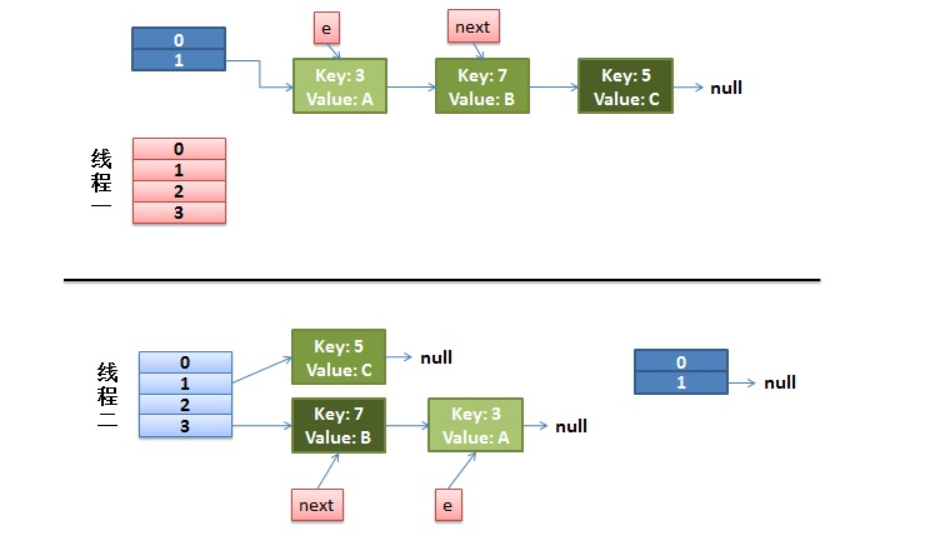1.先是执行 newTalbe[i] = e;

2.然后是e = next，导致了e指向了key(7)，

3.而下一次循环的next = e.next导致了next指向了key(3)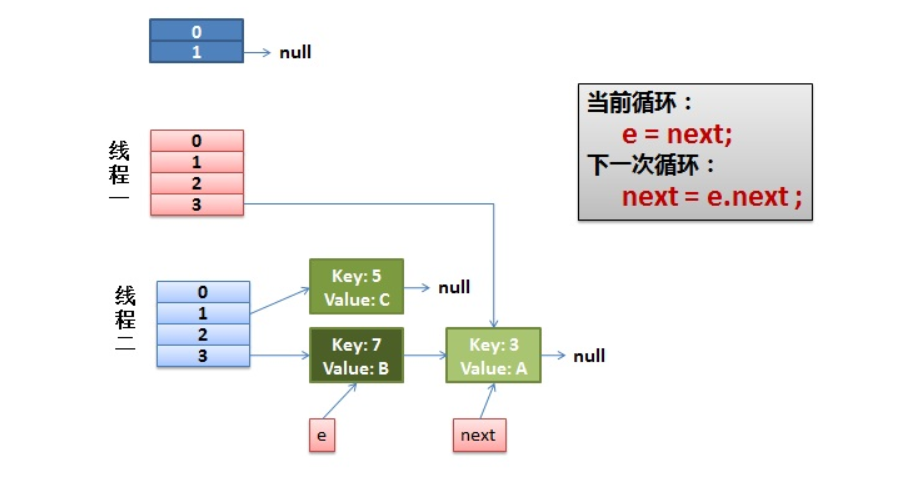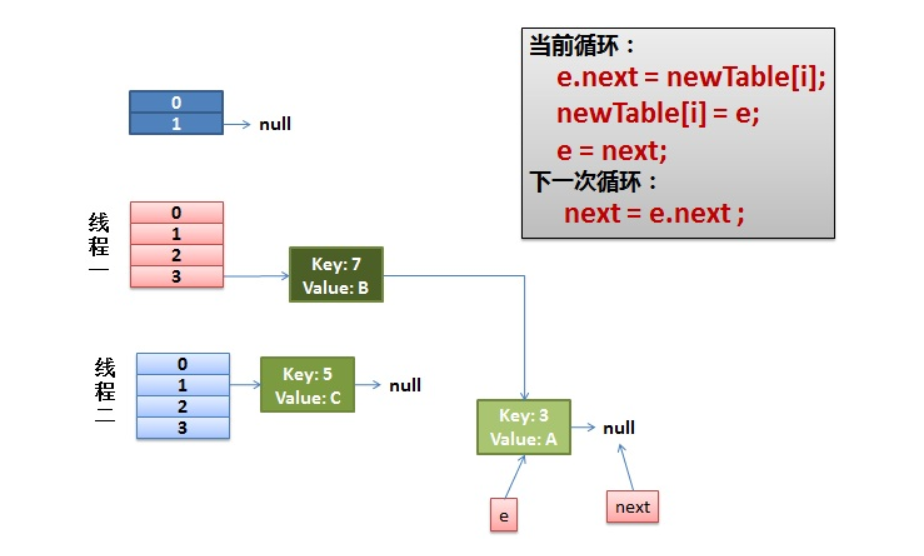e.next = newTable[i] 导致  key(3).next 指向了 key(7)posted @ 2018-08-13 21:44  妮蔻  阅读(...)  评论(...编辑  收藏